Next: B. Expressing the Equations Up: Appendix Previous: Appendix

# A. Re-expressing X1 as a Function of X2

In the first step the Hamiltonian (4.1) and the energy dispersion (4.4) are transformed into dimensionless units with the expressions:(7.1)

The energy dispersion then takes the following form: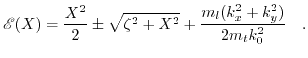(7.2)

Setting the determinant of the dimensionless Hamiltonian to zero allows to express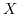as a function of energy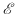: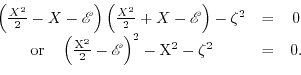(7.3)

Re-expressing the fourth order equation (7.3) as a second order equation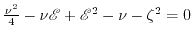by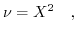(7.4)

we find the solution,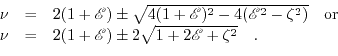(7.5)

This formulation preserves all four solutions for. Embracing two sets ofvalues in two separate equations leads to the following expressions: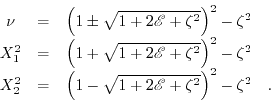(7.6)

Using the following identities: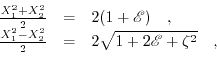(7.7)

leads to the desired expressions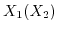and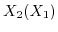.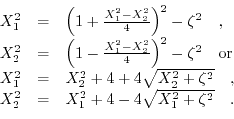(7.8)

The transformation to dimensionless units of the corresponding expression for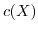is: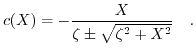(7.9)Next: B. Expressing the Equations Up: Appendix Previous: Appendix

T. Windbacher: Engineering Gate Stacks for Field-Effect Transistors